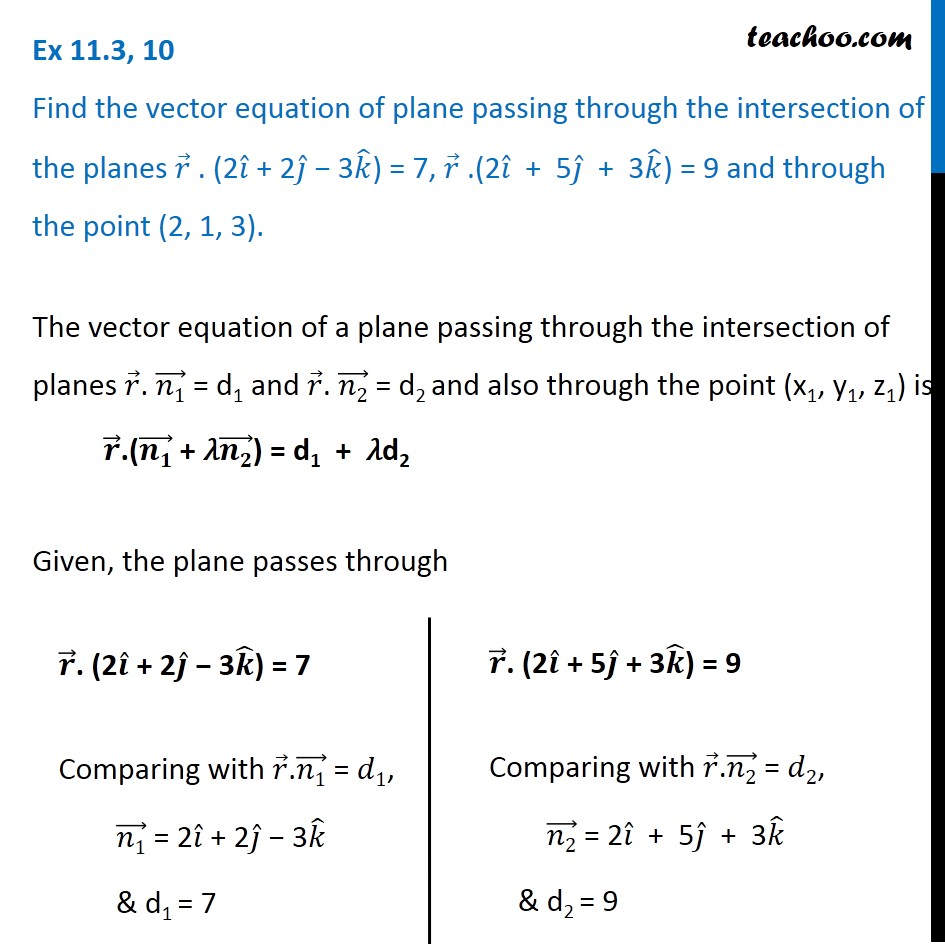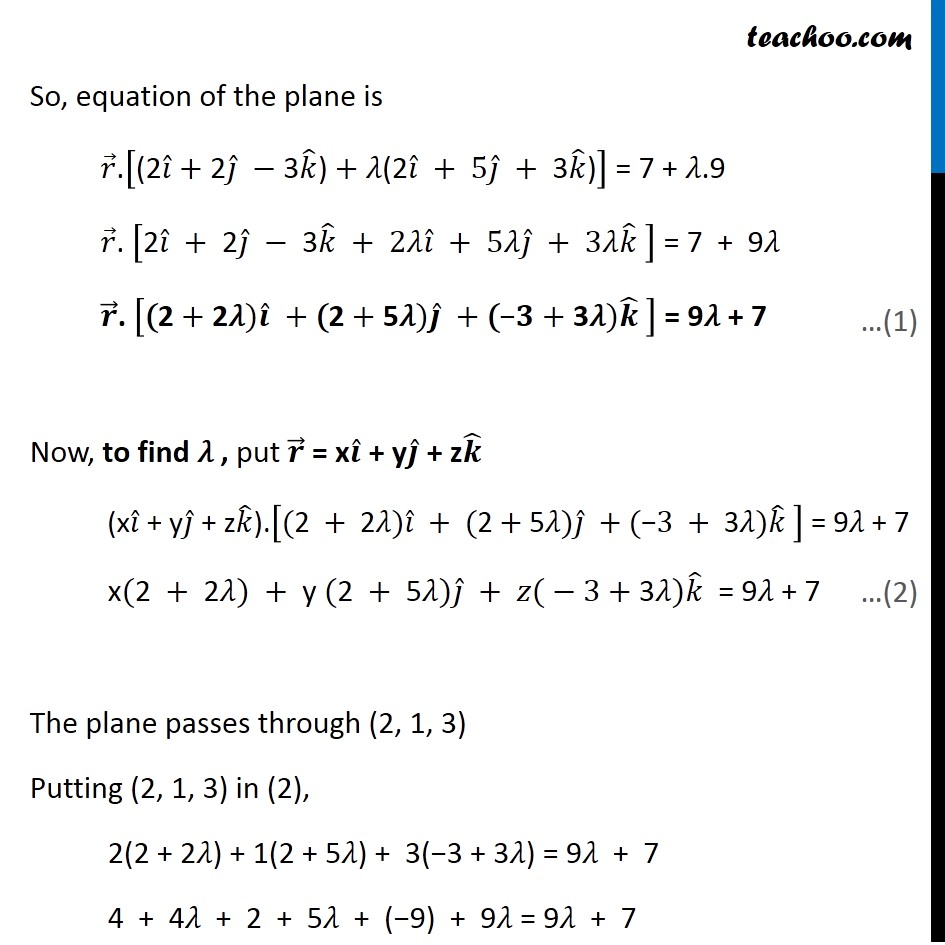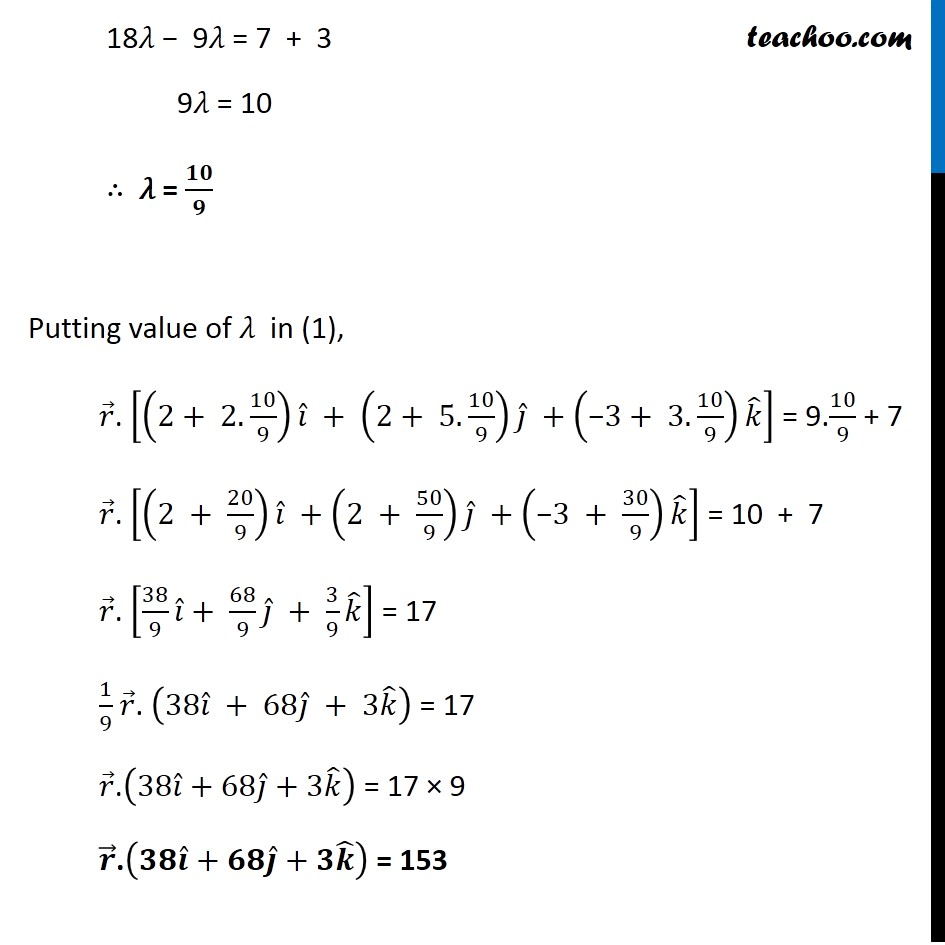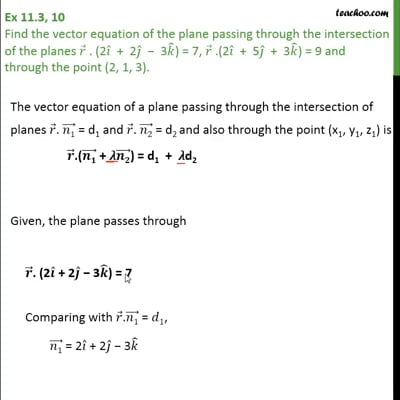Ex 11.3

Chapter 11 Class 12 Three Dimensional Geometry
Serial order wiseThis video is only available for Teachoo black users

Introducing your new favourite teacher - Teachoo Black, at only ₹83 per month

### Transcript

Ex 11.3, 10 Find the vector equation of plane passing through the intersection of the planes 𝑟 ⃗ . (2𝑖 ̂ + 2𝑗 ̂ − 3𝑘 ̂) = 7, 𝑟 ⃗ .(2𝑖 ̂ + 5𝑗 ̂ + 3𝑘 ̂) = 9 and through the point (2, 1, 3).The vector equation of a plane passing through the intersection of planes 𝑟 ⃗. (𝑛1) ⃗ = d1 and 𝑟 ⃗. (𝑛2) ⃗ = d2 and also through the point (x1, y1, z1) is 𝒓 ⃗.((𝒏𝟏) ⃗ + 𝜆(𝒏𝟐) ⃗) = d1 + 𝜆d2 Given, the plane passes through The vector equation of a plane passing through the intersection of planes 𝑟 ⃗. (𝑛1) ⃗ = d1 and 𝑟 ⃗. (𝑛2) ⃗ = d2 and also through the point (x1, y1, z1) is 𝒓 ⃗.((𝒏𝟏) ⃗ + 𝜆(𝒏𝟐) ⃗) = d1 + 𝜆d2 Given, the plane passes through 𝒓 ⃗. (2𝒊 ̂ + 2𝒋 ̂ − 3𝒌 ̂) = 7 Comparing with 𝑟 ⃗.(𝑛1) ⃗ = 𝑑1, (𝑛1) ⃗ = 2𝑖 ̂ + 2𝑗 ̂ − 3𝑘 ̂ & d1 = 7 𝒓 ⃗. (2𝒊 ̂ + 5𝒋 ̂ + 3𝒌 ̂) = 9 Comparing with 𝑟 ⃗.(𝑛2) ⃗ = 𝑑2, (𝑛2) ⃗ = 2𝑖 ̂ + 5𝑗 ̂ + 3𝑘 ̂ & d2 = 9 So, equation of the plane is 𝑟 ⃗.["(2" 𝑖 ̂+"2" 𝑗 ̂" " −"3" 𝑘 ̂")" +"𝜆(2" 𝑖 ̂" " + 5𝑗 ̂" " + "3" 𝑘 ̂")" ] = 7 + 𝜆.9 𝑟 ⃗. ["2" 𝑖 ̂" " +" 2" 𝑗 ̂" " − "3" 𝑘 ̂ + 2"𝜆" 𝑖 ̂ + 5"𝜆" 𝑗 ̂ + 3"𝜆" 𝑘 ̂ ] = 7 + 9"𝜆" 𝒓 ⃗. ["(2" +"2𝜆" )𝒊 ̂" " +"(2" +"5𝜆" )𝒋 ̂ +"(−" 𝟑+"3𝜆" )𝒌 ̂ ] = 9"𝜆" + 7 Now, to find 𝜆 , put 𝒓 ⃗ = x𝒊 ̂ + y𝒋 ̂ + z𝒌 ̂ (x𝑖 ̂ + y𝑗 ̂ + z𝑘 ̂).["(2 " + "2𝜆" )𝑖 ̂" " + "(2" +"5𝜆" )𝑗 ̂ +"(−" 3" " +" 3𝜆" )𝑘 ̂ ] = 9𝜆 + 7 x"(2 "+" 2𝜆")" "+ "y (2 "+" 5𝜆")𝑗 ̂ + 𝑧"("−3+"3𝜆")𝑘 ̂ = 9𝜆 + 7 The plane passes through (2, 1, 3) Putting (2, 1, 3) in (2), 2(2 + 2𝜆) + 1(2 + 5𝜆) + 3(−3 + 3𝜆) = 9𝜆 + 7 4 + 4𝜆 + 2 + 5𝜆 + (−9) + 9𝜆 = 9𝜆 + 7 18𝜆 − 9𝜆 = 7 + 3 9𝜆 = 10 ∴ 𝜆 = 𝟏𝟎/𝟗 Putting value of 𝜆 in (1), 𝑟 ⃗. [(2+ 2. 10/9) 𝑖 ̂ + (2+ 5. 10/9) 𝑗 ̂ +("−" 3+ 3. 10/9) 𝑘 ̂ ] = 9.10/9 + 7 𝑟 ⃗. [(2 + 20/9) 𝑖 ̂ +(2 + 50/9) 𝑗 ̂ +("−" 3 + 30/9) 𝑘 ̂ ] = 10 + 7 𝑟 ⃗. [38/9 𝑖 ̂+ 68/9 𝑗 ̂ + 3/9 𝑘 ̂ ] = 17 1/9 𝑟 ⃗. (38𝑖 ̂ + 68𝑗 ̂ + 3𝑘 ̂ ) = 17 𝑟 ⃗.(38𝑖 ̂+68𝑗 ̂+3𝑘 ̂ ) = 17 × 9 𝒓 ⃗.(𝟑𝟖𝒊 ̂+𝟔𝟖𝒋 ̂+𝟑𝒌 ̂ ) = 153